# Test: Time Domain Analysis of Control Systems

## 10 Questions MCQ Test GATE ECE (Electronics) 2023 Mock Test Series | Test: Time Domain Analysis of Control Systems

Description
Attempt Test: Time Domain Analysis of Control Systems | 10 questions in 30 minutes | Mock test for Electrical Engineering (EE) preparation | Free important questions MCQ to study GATE ECE (Electronics) 2023 Mock Test Series for Electrical Engineering (EE) Exam | Download free PDF with solutions
QUESTION: 1

### The solution of the differential equation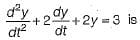Solution:

Taking Laplace transform on both sides of the given differential equation, we get (s2 + 2s + 2) Y(s) = 3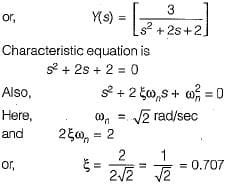Since, ξ < 1, therefore given system is underdamped.

QUESTION: 2

### A second order system is said to be critically damped if the damping factor (ξ) is

Solution:

If ξ < 1, system is underdamped.
If ξ > 1, system is overdamped.
If ξ= 1, system is critically damped.

QUESTION: 3

### The ratio of damped frequency to natural frequency of the given system having damping ratio ξ is

Solution: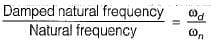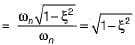QUESTION: 4

In time domain specification, the time delay is the time required for the response to reach

Solution:

Delay time (td) is the time required for the response curve to reach 50% of the final value.

QUESTION: 5

For the second order prototype system, when the undamped natural frequency increases, the maximum overshoot of the output

Solution:

Undamped natural frequency = ωn and maximum overshoot,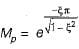Since Mp is independent of ωn, therefore with increase in ωn, Mp remains constant

QUESTION: 6

Damping in a control system is a function of

Solution:

The general relation between ξ and gain K is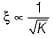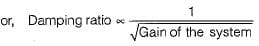QUESTION: 7

Damping ratio is defined as the ratio of

Solution: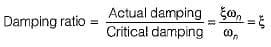QUESTION: 8

A linear second-order system with the transfer function G(s) =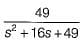is initially at rest and is subjected to a step input signal.
The response of the system will exhibit a peak overshoot of

Solution: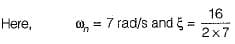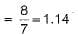Since ξ > 1, therefore the system is overdamped. Hence, there will be no peak overshoot in the output response of the system.

QUESTION: 9

The closed-loop transfer function of a system is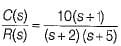Which of the following statements is not true?

Solution: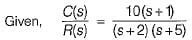It’s characteristic equation is (s + 2) (s + 5) = 0
or, s2 + 7s +10 = 0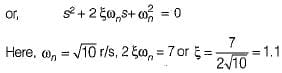Thus, damping ratio ξ > 1.
Hence, option (c) is false.

QUESTION: 10

The steady state error of a control system can be reduced by

Solution:

Time constant of the system,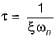When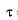is increased means damping is reduced, thus steady state error reduces.
Also, when gain of the system increases, then damping reduces and therefore, steady state error reduces.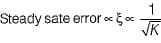Use Code STAYHOME200 and get INR 200 additional OFF Use Coupon Code